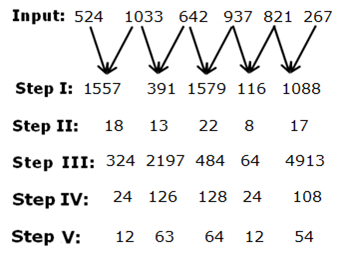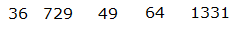# SBI Clerk Mains Reasoning (Day-77)

Dear Aspirants, Our IBPS Guide team is providing new series of Reasoning Questions for SBI Clerk Mains 2020 so the aspirants can practice it on a daily basis. These questions are framed by our skilled experts after understanding your needs thoroughly. Aspirants can practice these new series questions daily to familiarize with the exact exam pattern and make your preparation effective.

Start Quiz

Ensure Your Ability Before the ExamTake SBI Clerk 2020 Mains Free Mock Test

Month with date based puzzle

Direction(1-5): Study the following information carefully and answer the questions given below:

Ten persons Q, F, R, T, B, C, M, K, G and Z have seminars in January, May, June, April and March with two seminars in each month. The seminar is scheduled on 9th and 16th of the month. Each one of them is in different age 27, 29, 52, 36, 39, 12, 15, 18, 43 and 24. All the above information is not necessarily in the same order.

G does not attend the seminar before M. T attends the seminar in April month. Only one person attends the seminar between T and F. The persons who attend the seminar on January 16 will be twice the age of Z. M attends the seminar in May month. Only one person attends the seminar between M and G. G is 4 years elder than B. The one who is the eldest attends the seminar immediately after B. Two persons attend theseminar between the one who is the eldest and R. C attends the seminar before K. The number of personsattend the seminar before K is same as the number of personsattend the seminar after Q. K’s age is a prime number.The age of one who attends the seminar on January 9th is not an odd number. T’s age is twice that of F’s age. Z does not attend the seminar in a month which has less than 31 days. Z is 3 years younger than M.

1) Who among the following person’s age is 27?

A) F

B) B

C) Q

D) R

E) None of these

2) How many persons attend the seminar between F and the one whose age 29?

A) 1

B) 5

C) 3

D) 4

E) None of these

3) Who attends the seminar on May 9th?

A) F-36

B) M-15

C) C-52

D) F-24

E) None of these

4) Four of the following five are alike in a certain way and hence they form a group. Which one of the following does not belong to that group?

A) G

B) The one whose age is 15

C) The one whose age is 27

D) T

E) Q

5) If Z is related to 24, T is related to 39, in the same way, K is related to which of the following?

A) 15

B) 24

C) 43

D) 36

D) None of these

Input output

Directions (6-10): Study the following information to answer the given questions.

A number arrangement machine when given an input line of numbers rearranges them following a particular rule. The following is an illustration of input and re-arrangement.Step V is the output of the above input.

As per the rules followed in the above steps, find out in each of the following questions the appropriate steps for the given input.

Input:  317   823   967    1047    1127    198

6) What is the sum of the second element from the right end in step I and the second element from the left end in step V?

A) 121

B) 143

C) 169

D) 153

E) None of these

7) Which of the following element is immediate left of second to the right of the element which is third from the left end in step IV?

A) 108

B) 126

C) 128

D) 24

E) None of these

8) How many elements are there between ‘126’ and ‘9’ in step IV?

A) 3

B) 5

C) 2

D) 4

E) None of these

9) Which of the following step represents the following output?A) Step III

B) Step II

C) Step IV

D) There is no such step

WNone of these

10) What is the position of “36” in step III from the left end?

A) Fifth

B) Second

C) Fourth

D) Third

E) First

Directions (1-5) :G is 4 years elder than B.

B+4=G ( there is only one possible case 39 and 43)

G=43 and B=39

Z is 3 years younger than M.

Z=M-3

Possible cases are (12,15),(15,18),(24,27) and (36,39)

The persons who attend the seminar on January 16 will be twice the age of Z. (There is only one case satisfy the above statement 12 and 15)

Hence,Z=12 and M=15

The age of the person who attends a seminar on Jan 16th will be 24.

K’s age is a prime number (29 and 43(is already fixed for G)). So K’s age is 29.

T’s age is twice that of F’s age.

T=2F(Now there is only one possible case)

T=36

F=18

T attends the seminar in April month.Only one person attends the seminar between T and F. Only one person attends the seminar between M and G. M attends the seminar in May month. G does not attend the seminar before M. The age of the person who attends a seminar on Jan 16th will be 24. The one who is the eldestattends the seminar immediately after B. Two persons attend the seminar between the one who is the eldest and R. Z does not attend the seminar in a month which has less than 31 days. C attends the seminar before K. The number of persons attend the seminar before K is same as the number of persons attend the seminar after Q. The age of one who attends the seminar on January 9th is not an odd number.

If T attends the seminar on April 9.Directions (6-10):

Step I:  Alternative addition and subtraction of two numbers which is represented by the arrow.

Step II:  Sum of the digits obtained in a number

Step III:  Square of an even number and cube of an odd number

Step IV:  Multiplication of each digit in a number

Step V:  Each number is divided by 2